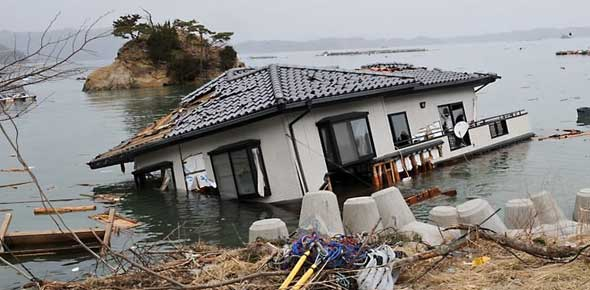# Final Exam Review Part 2

40 Questions | Total Attempts: 53Settings• 1.
List the eons from oldest to youngest.
• A.

• B.

• C.

• D.

• 2.
Oceanic lithosphere that is old will subduct at 90 degree angles.
• A.

True

• B.

False

• 3.
Put these three Earthquakes in order of smallest to largest amount of energy released.
• A.

Haiti, Chile, Japan

• B.

Haiti, Japan, Chile

• C.

Japan, Chile, Haiti

• D.

Chile, Japan, Haiti

• 4.
What is the most abundant mineral on Earth’s surface?
• A.

Quartz

• B.

Feldspars

• C.

Muscovite

• D.

Olivine

• 5.
Which of these is a trigger of mass wasting?
• A.

• B.

Earthquakes

• C.

Water

• D.

B and c

• 6.
A ridge axis is elevated when spreading rates are low.
• A.

True

• B.

False

• 7.
Which era means “recent life”?
• A.

Paleozoic

• B.

Cenozoic

• C.

Mesozoic

• D.

Precambriazoic

• 8.
Which of these geologic events happened in the Mesozoic era?
• A.

Pangaea began to break apart

• B.

Pangaea assembled

• C.

Cratons were created

• D.

Eastern and Western margins of North America began to differ from each other

• 9.
Subduction of a lithospheric plate occurs at which type of plate boundary?
• A.

Divergent

• B.

Tranform

• C.

Oceanic-continental

• D.

Continental-continental

• 10.
Which of the following comprises soil?
• A.

Humus

• B.

Water

• C.

Air

• D.

All of the above

• 11.
What is the mathematical law that describes groundwater flow?
• A.

Darcy’s law

• B.

• C.

Pythagorean theorem

• D.

Theory of Relativity

• 12.
The primary means of heat transfer within Earth is:
• A.

Mantle convection

• B.

Mantle conduction

• C.

The outer core

• D.

The Sun

• 13.
You will find massive sediment accumulation on:
• A.

Ocean trenches

• B.

Abyssal plains

• C.

Oceanic ridges

• D.

Guyots

• 14.
How easily water can move through a rock:
• A.

Porosity

• B.

Permeability

• 15.
Which of the following factors does not affect rock strength?
• A.

Time

• B.

Temperature

• C.

Rock type

• D.

Igneous texture

• 16.
When broad upwarps in basement rock deform the overlying cover of sedimentary strata and generate large folds. When upwarping produces a circular or slightly elongated structure this is called a ______.
• A.

Dome

• B.

Anticline

• C.

Basin

• D.

Syncline

• 17.
These surface waves move in a ripple motion.
• A.

P waves

• B.

S waves

• C.

L waves

• D.

R waves

• 18.
The most important factor driving metamorphism is:
• A.

Pressure

• B.

Chemically active fluids

• C.

Heat

• D.

Foliation

• 19.
Volcanoes can be found at the following plate boundaries except:
• A.

Divergent

• B.

Oceanic-oceanic convergent

• C.

Oceanic-continental convergent

• D.

Transform fault

• 20.
The last step that a scientist will take is:
• A.

Observe natural events

• B.

Theorize

• C.

Hypothesize

• D.

Collect data

• 21.
Two minerals with exactly the same chemical composition cannot have different internal structures:
• A.

True

• B.

False

• 22.
An example of a concordant igneous intrusion is a:
• A.

Pluton

• B.

Dike

• C.

Batholith

• D.

Sill

• 23.
This type of volcano has a very simple, distinctive shape determined by the slope that loose pyroclastic material maintains as it comes to rest.
• A.

Startovolcano

• B.

Shield

• C.

Cinder cone

• D.

Caldera

• 24.
Minerals are highly segregated and give the rock a banded appearance in this foliated texture.
• A.

Schistosity

• B.

Gneissic

• C.

Slatey

• D.

Rock

• 25.
Which of these is not a greenhouse gas? a) nitrogen   b) CO2   c) methane   d) water vapor
• A.

Nitrogen

• B.

CO2

• C.

Methane

• D.

Water vapor

Related TopicsBack to top